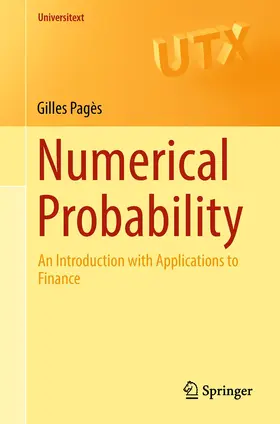# PagèsNumerical Probability

An Introduction with Applications to Finance

1. Auflage 2018, 579 Seiten, Kartoniert, Paperback, Format (B × H): 159 mm x 237 mm, Gewicht: 902 g Reihe: Universitext
ISBN: 978-3-319-90274-6
Verlag: Springer, Berlin

## PagèsNumerical Probability

This textbook provides a self-contained introduction to numerical methods in probability with a focus on applications to finance.

Topics covered include the Monte Carlo simulation (including simulation of random variables, variance reduction, quasi-Monte Carlo simulation, and more recent developments such as the multilevel paradigm), stochastic optimization and approximation, discretization schemes of stochastic differential equations, as well as optimal quantization methods. The author further presents detailed applications to numerical aspects of pricing and hedging of financial derivatives, risk measures (such as value-at-risk and conditional value-at-risk), implicitation of parameters, and calibration.

Aimed at graduate students and advanced undergraduate students, this book contains useful examples and over 150 exercises, making it suitable for self-study.

Graduate

## Weitere Infos & Material

1 Simulation of random variables.- 2 The Monte Carlo method and applications to option pricing.- 3 Variance reduction.- 4 The Quasi-Monte Carlo method.- 5 Optimal Quantization methods I: cubatures.- 6 Stochastic approximation with applications to finance.- 7 Discretization scheme(s) of a Brownian diffusion.- 8 The diffusion bridge method: application to path-dependent options (II).- 9 Biased Monte Carlo simulation, Multilevel paradigm.- 10 Back to sensitivity computation.- 11 Optimal stopping, Multi-asset American/Bermuda Options.- 12 Miscellany.

Pagès, Gilles
Gilles Pagès is full Professor of Mathematics at Sorbonne Université (formerly Université Pierre & Marie Curie) specializing in probability theory, numerical probability and mathematical finance. He was the director of the Laboratoire de Probabiliéts & Modèles Aéatoires (now Laboratoire de Probabilités, Statistique et Modélisation) from 2009 to 2014, and has been the director of the Master 2 "Probabilités & Finance", also known as "Master ElKaroui", since 2001. He has published over 100 research articles in probability theory, numerical probability and financial modelling. He is also the author of several graduate and undergraduate textbooks in statistics, applied probability and mathematical finance.

WIR VERWENDEN COOKIES

Einige Cookies sind notwendig für den Betrieb der Seite, während andere uns helfen, Ihnen ein optimales Erlebnis unserer Webseite zu ermöglichen.